# Overland Flow

In this post, we'll look at overland flow -- how rainwater drains across a landscape. The equations of motion are pretty rowdy and have some fascinating effects. To derive them, we'll start from the shallow water or Saint Venant equations for the water layer thickness $h$ and velocity $u$:

\begin{align} \frac{\partial h}{\partial t} + \nabla\cdot hu & = \dot a \\ \frac{\partial}{\partial t}hu + \nabla\cdot hu\otimes u & = -gh\nabla (b + h) - k|u|u \end{align}

The final term in the momentum equation represents frictional dissipation and $k$ is a (dimensionless) friction coefficient. Using the shallow water equations for predicting overland flow is challenging because the thickness can go to zero.

For many thin open channel flows, however, the fluid velocity can be expressed purely in terms of the surface slope and other factors. You could arrive at one such simplification by assuming that the inertial terms in the momentum equation are zero:

$$k|u|u + gh\nabla(b + h) = 0.$$

This approximation is known as the Darcy-Weisbach equation. We'll use it in the following because it's simple and it illustrates all the major difficulties. For serious work, you'd probably want to use the Manning formula, as it has some theoretical justification for turbulent open channel flows. The overall form of the equation and the resulting numerical challenges are the same in each case.

Putting together the Darcy-Weisbach equation for the velocity with the mass conservation equation gives a single PDE for the water layer thickness:

$$\frac{\partial h}{\partial t} - \nabla\cdot\left(\sqrt{\frac{gh^3}{k}}\frac{\nabla(b + h)}{\sqrt{|\nabla(b + h)|}}\right) = \dot a.$$

This looks like a parabolic equation, but there's a catch! The diffusion coefficient is proportional to $h^{3/2}$, so it can go to zero when $h = 0$; all the theory for elliptic and parabolic equations assumes that the diffusion coefficient is bounded below. For a non-degenerate parabolic PDE, disturbances propagate with infinite speed. For the degenerate problem we're considering, that's no longer true -- the $h = 0$ contour travels with finite speed! While we're using the Darcy-Weisbach equation to set the velocity here, we still get finite propagation speed if we use the Manning equation instead. What's important is that the velocity is propertional to some power of the thickness and surface slope.

Eliminating the velocity entirely from the problem is convenient for analysis, but not necessarily the best way to go numerically. We'll retain the velocity as an unknown, which gives the resulting variational form much of the same character as the mixed discretization of the heat equation.

As our model problem, we'll use the dam break test case from Santillana and Dawson (2009). They discretized the overland flow equations using the local discontinuous Galerkin or LDG method, which extends DG for hyperbolic systems to mixed advection-diffusion problems. We'll use different numerics because Firedrake has all the hipster elements. I'm eyeballing the shape of the domain from their figures.

import numpy as np
import pygmsh

geometry = pygmsh.built_in.Geometry()

coords = np.array(
[
[0.0, 0.0],
[3.0, 0.0],
[3.0, 2.0],
[2.0, 2.0],
[2.0, 4.0],
[3.0, 4.0],
[3.0, 6.0],
[0.0, 6.0],
[0.0, 4.0],
[1.0, 4.0],
[1.0, 2.0],
[0.0, 2.0],
]
)

points = [
np.column_stack((coords, np.zeros(len(coords))))
]
edges = [
geometry.add_line(p1, p2) for p1, p2 in
zip(points, points[1:] + [points])
]

with open("dam.geo", "w") as geo_file:
geo_file.write(geometry.get_code())

!gmsh -2 -format msh2 -v 0 -o dam.msh dam.geo

import firedrake

mesh = firedrake.Mesh("dam.msh")

import matplotlib.pyplot as plt

fig, ax = plt.subplots()
ax.set_aspect("equal")
firedrake.triplot(mesh, axes=ax);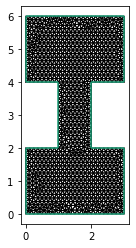The bed profile consists of an upper, elevated basin, together with a ramp down to a lower basin.

from firedrake import Constant, min_value, max_value

x = firedrake.SpatialCoordinate(mesh)

y_0 = Constant(2.0)
y_1 = Constant(4.0)
b_0 = Constant(0.0)
b_1 = Constant(1.0)
b_expr = b_0 + (b_1 - b_0) * max_value(0, min_value(1, (x - y_0) / (y_1 - y_0)))

S = firedrake.FunctionSpace(mesh, "CG", 1)
b = firedrake.interpolate(b_expr, S)

fig = plt.figure()
axes.set_box_aspect((3.0, 6.0, 1.0))
axes.set_axis_off()
firedrake.trisurf(b, axes=axes);As I alluded to before, rather than eliminate the velocity entirely, we'll keep it as a field to be solved for explicitly. The problem we're solving, while degenerate, is pretty similar to the mixed form of the heat equation. This suggests that we should use element pairs that are stable for mixed Poisson. Here I'm using the MINI element: continuous linear basis functions for the thickness, and continuous linear enriched with cubic bubbles for the velocity. We could also have used a more proper $H(\text{div})$-conforming pair, like discontinuous Galerkin for the thickness and Raviart-Thomas or Brezzi-Douglas-Marini for the velocity.

cg1 = firedrake.FiniteElement("CG", "triangle", 1)
Q = firedrake.FunctionSpace(mesh, cg1)
b3 = firedrake.FiniteElement("B", "triangle", 3)
V = firedrake.VectorFunctionSpace(mesh, cg1 + b3)
Z = Q * V


The dam break problem specifies that the thickness is equal to 1 in the upper basin and 0 elsewhere. I've done a bit of extra work below because the expression for $h$ is discontinuous, and interpolating it directly gives some obvious mesh artifacts. Instead, I've chosen to project the expression and clamp it above and below.

h_expr = firedrake.conditional(x >= y_1, 1.0, 0.0)
h_0 = firedrake.project(h_expr, Q)
h_0.interpolate(min_value(1, max_value(0, h_0)));

fig, ax = plt.subplots()
ax.set_aspect("equal")
colors = firedrake.tripcolor(h_0, axes=ax)
fig.colorbar(colors);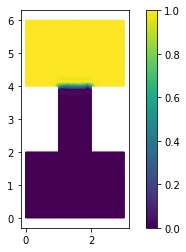z = firedrake.Function(Z)
z_n = firedrake.Function(Z)
δt = Constant(1.0 / 32)
z_n.sub(0).assign(h_0)
z.sub(0).assign(h_0);


The test case in the Santillana and Dawson paper uses a variable friction coefficient in order to simulate the effect of increased drag when flowing over vegetation.

from firedrake import inner

k_0 = Constant(1.0)
δk = Constant(4.0)
r = Constant(0.5)
x_1 = Constant((1.5, 1.0))
x_2 = Constant((1.0, 3.5))
x_3 = Constant((2.0, 2.5))
ψ = sum(
[
max_value(0, 1 - inner(x - x_i, x - x_i) / r**2)
for x_i in [x_1, x_2, x_3]
]
)
k = k_0 + δk * ψ

fig, axes = plt.subplots()
axes.set_aspect("equal")
firedrake.tripcolor(firedrake.interpolate(k, S), axes=axes);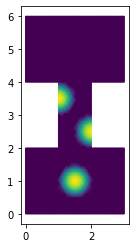The code below defines the variational form of the overland flow equations.

from firedrake import div, grad, dx

g = Constant(1.0)

h, q = firedrake.split(z)
h_n = firedrake.split(z_n)
ϕ, v = firedrake.TestFunctions(Z)

F_h = ((h - h_n) / δt + div(q)) * ϕ * dx
friction = k * inner(q, q)**0.5 * q
gravity = -g * h**3 * grad(b + h)
F_q = inner(friction - gravity, v) * dx
F = F_h + F_q


We'll run into trouble if we try and use a Newton-type method on the true variational form. Notice how the $q$-$q$ block of the derivative will go to zero whenever $q = 0$. This will happen whenever the thickness is zero too. The usual hack is to put a fudge factor $\varepsilon$ into the variational form, as shown below.

ϵ = Constant(1e-3)
friction = k * (inner(q, q) + ϵ**2)**0.5 * q
gravity = -g * h**3 * grad(b + h)
F_qϵ = inner(friction - gravity, v) * dx
F_ϵ = F_h + F_qϵ


The disadvantage of is that we're then solving a slightly different physics problem. We don't have a great idea ahead of time of what $\varepsilon$ should be either. If we choose it too large, the deviation from the true problem is large enough that we can't believe the results. But if we choose it too small, the derivative will fail to be invertible.

We can take a middle course by instead just using the perturbed variational form just to define the derivative in Newton's method, but keep the true variational form as the quantity to find a root for. To do this, we'll pass the derivative of $F_\varepsilon$ as the Jacobian or J argument to the nonlinear variational problem object. Choosing $\varepsilon$ too small will still cause the solver to crash. Taking it to be too large, instead of causing us to solve a completely different problem, will now only make the solver go slower instead. We still want to make $\varepsilon$ as small as possible, but to my mind, getting the right answer slowly is a more tolerable failure mode than getting the wrong answer.

bcs = firedrake.DirichletBC(Z.sub(1), firedrake.zero(), "on_boundary")
J = firedrake.derivative(F_ϵ, z)
problem = firedrake.NonlinearVariationalProblem(F, z, bcs, J=J)


We'll have one final difficulty to overcome -- what happens if the thickness inadvertently becomes negative? There's a blunt solution that everyone uses, which is to clamp the thickness to 0 from below at every step. Clamping can work in many cases. But if you're using a Runge-Kutta method, it only assures positivity at the end of each step and not in any of the intermediate stages. We can instead formulate the whole problem, including the non-negativity constraint, as a variational inequality. Much like how some but not all variational problems arise from minimization principles, some variational inequalities arise from minimization principles with inequality constraints, like the obstacle problem. But variational inequalities are a more general class of problem than inequality-constrained minimization. Formulating overland flow as as a variational inequality is a bit of overkill for the time discretization that we're using. Nonetheless, I'll show how to do that in the following just for illustrative purposes. We first need two functions representing the upper and lower bounds for the solution. In this case, the upper bound is infinity.

from firedrake.petsc import PETSc

upper = firedrake.Function(Z)
with upper.dat.vec as upper_vec:
upper_vec.set(PETSc.INFINITY)


The thickness is bounded below by 0, but there's no lower bound at all on the flux, so we'll set only the flux entries to negative infinity.

lower = firedrake.Function(Z)
with lower.sub(1).dat.vec as lower_vec:
lower_vec.set(PETSc.NINFINITY)


When we want to solve variational inequalities, we can't use the usual Newton solvers in PETSc -- we have a choice between a semi-smooth Newton (vinewtonssls) and an active set solver (vinewtonrsls). I couldn't get the semi-smooth Newton solver to work and I have no idea why.

params = {
"solver_parameters": {
"snes_type": "vinewtonrsls",
"ksp_type": "gmres",
"pc_type": "lu",
}
}

solver = firedrake.NonlinearVariationalSolver(problem, **params)


Finally, we'll run the timestepping loop. Here we pass the bounds explicitly on each call to solve.

import tqdm

final_time = 60.0
num_steps = int(final_time / float(δt))

hs = [z.sub(0).copy(deepcopy=True)]
qs = [z.sub(1).copy(deepcopy=True)]

for step in tqdm.trange(num_steps):
solver.solve(bounds=(lower, upper))
z_n.assign(z)

h, q = z.split()
hs.append(h.copy(deepcopy=True))
qs.append(q.copy(deepcopy=True))

100%|██████████| 1920/1920 [08:22<00:00,  3.82it/s]


Movie time as always.

%%capture

from matplotlib.animation import FuncAnimation

fig, axes = plt.subplots()
axes.set_aspect("equal")
axes.get_xaxis().set_visible(False)
axes.get_yaxis().set_visible(False)

colors = firedrake.tripcolor(
hs, axes=axes, vmin=0, vmax=1.0, cmap="Blues", num_sample_points=4
)
fn_plotter = firedrake.FunctionPlotter(mesh, num_sample_points=4)

def animate(h):
colors.set_array(fn_plotter(h))

interval = 1e3 / 60
animation = FuncAnimation(fig, animate, frames=hs, interval=interval)

from IPython.display import HTML

HTML(animation.to_html5_video())


As some a posteriori sanity checking, we can evaluate how much the total water volume deviates.

volumes = np.array([firedrake.assemble(h * dx) for h in hs])
volume_error = (volumes.max() - volumes.min()) / volumes.mean()
print(f"Volume relative error: {volume_error:5.2g}")

Volume relative error: 0.013


Where a truly conservative scheme would exactly preserve the volume up to some small multiple of machine precision, we can only get global conservation up to the mesh resolution with our scheme. Instead, there are spurious "sources" at the free boundary. Likewise, there can be spurious sinks in the presence of ablation, so the sign error can go either way. This topic is covered in depth in this paper.

fig, axes = plt.subplots()
ts = np.linspace(0.0, final_time, num_steps + 1)
axes.set_xlabel("time")
axes.set_ylabel("volume ($m^3$)")
axes.plot(ts, volumes);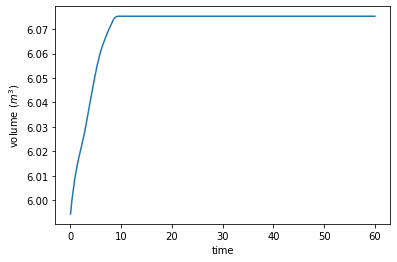We can examine the fluxes after the fact in order to see where the value of $\varepsilon$ that we picked sits.

qms = [firedrake.project(inner(q, q)**0.5, Q) for q in qs]
area = firedrake.assemble(Constant(1) * dx(mesh))
qavgs = np.array([firedrake.assemble(q * dx) / area for q in qms])
print(f"Average flux: {qavgs.mean()*100**2:5.1f} cm²/s")
print(f"Fudge flux:   {float(ϵ)*100**2:5.1f} cm²/s")

Average flux: 266.5 cm²/s
Fudge flux:    10.0 cm²/s


The fudge flux is 1/25 that of the average. This is quite a bit smaller, but not so much so that we should feel comfortable with this large a perturbation to the physics equations themselves. The ability to use it only in the derivative and not in the residual is a huge help.

To wrap things up, the overland flow equations are a perfect demonstration of how trivially equivalent forms of the same physical problem can yield vastly different discretizations. Writing the system as a single parabolic PDE might seem simplest, but there are several potential zeros in the denominator that require some form of regularization. By contrast, using a mixed form introduces more unknowns and a nonlinear equation for the flux, but there's wiggle room within that nonlinear equation. This makes it much easier to come up with a robust solution procedure, even if it includes a few uncomfortable hacks like using a different Jacobian from that of the true problem. Finally, while our discretization still works ok with no positivity constraint, PETSc has variational inequality solvers that make it possible to enforce positivity.

# Billiards on surfaces

In the previous post, I showed how to integrate Hamiltonian systems

\begin{align} \dot q & = +\frac{\partial H}{\partial p} \\ \dot p & = -\frac{\partial H}{\partial q} \end{align}

using methods that approximately preserve the energy. Here I'd like to look at what happens when there are non-trivial constraints

$$g(q) = 0$$

on the configuration of the system. The simplest example is the pendulum problem, where the position $x$ of the pendulum is constrained to lie on the circle of radius $L$ centered at the origin. These constraints are easy to eliminate by instead working with the angle $\theta$. A more complicated example is a problem with rotational degrees of freedom, where the angular configuration $Q$ is a 3 $\times$ 3 matrix. The constraint comes from the fact that this matrix has to be orthogonal:

$$Q^\top Q = I.$$

We could play similar tricks to the case of the pendulum and use Euler angles, but these introduce other problems when used for numerics. For this or other more complex problems, we'll instead enforce the constraints using a Lagrange multiplier $\lambda$, and working with the constrained Hamiltonian

$$H' = H - \lambda\cdot g(q).$$

We're then left with a differential-algebraic equation:

\begin{align} \dot q & = +\frac{\partial H}{\partial p} \\ \dot p & = -\frac{\partial H}{\partial q} + \lambda\cdot\nabla g \\ 0 & = g(q). \end{align}

If you feel like I pulled this multiplier trick out of a hat, you might find it more illuminating to think back to the Lagrangian formulation of mechanics, which corresponds more directly with optimization via the stationary action principle. Alternatively, you can view the Hamiltonian above as the limit of

$$H_\epsilon' = H + \frac{|p_\lambda|^2}{2\epsilon} - \lambda\cdot g(q)$$

as $\epsilon \to 0$, where $p_\lambda$ is a momentum variable conjugate to $\lambda$. This zero-mass limit is a singular perturbation, so actually building a practical algorithm from this formulation is pretty awful, but it can be pretty helpful conceptually.

For now we'll assume that the Hamiltonian has the form

$$H = \frac{1}{2}p^*M^{-1}p + U(q)$$

for some mass matrix $M$ and potential energy $U$. The 2nd-order splitting scheme to solve Hamilton's equations of motion in the absence of any constraints are

\begin{align} p_{n + \frac{1}{2}} & = p_n - \frac{\delta t}{2}\nabla U(q_n) \\ q_{n + 1} & = q_n + \delta t\cdot M^{-1}p_{n + \frac{1}{2}} \\ p_{n + 1} & = p_{n + \frac{1}{2}} - \frac{\delta t}{2}\nabla U(q_{n + 1}). \end{align}

To enforce the constraints, we'll add some extra steps where we project back onto the surface or, in the case of the momenta, onto its cotangent space. In the first stage, we solve the system

\begin{align} p_{n + \frac{1}{2}} & = p_n - \frac{\delta t}{2}\left\{\nabla U(q_n) - \lambda_{n + 1}\cdot \nabla g(q_n)\right\} \\ q_{n + 1} & = q_n - \delta t\cdot M^{-1}p_{n + \frac{1}{2}} \\ 0 & = g(q_{n + 1}). \end{align}

If we substitute the formula for $p_{n + 1/2}$ into the second equation and then substitute the resulting formula for $q_{n + 1}$ into the constraint $0 = g(q_{n + 1})$, we get a nonlinear system of equations for the new Lagrange multiplier $\lambda_{n + 1}$ purely in terms of the current positions and momenta. Having solved this nonlinear system, we can then substitute the value of $\lambda_{n + 1}$ to obtain the values of $p_{n + 1/2}$ and $q_{n + 1}$. Next, we compute the momentum at step $n + 1$, but subject to the constraint that it has to lie in the cotangent space of the surface:

\begin{align} p_{n + 1} & = p_{n + \frac{1}{2}} - \frac{\delta t}{2}\left\{\nabla U(q_{n + 1}) - \mu_{n + 1}\cdot \nabla g(q_{n + 1})\right\} \\ 0 & = \nabla g(q_{n + 1})\cdot M^{-1}p_{n + 1}. \end{align}

Once again, we can substitute the first equation into the second to obtain a linear system for the momentum-space multiplier $\mu$. Having solved for $\mu$, we can then back-substitute into the first equation to get $p_{n + 1}$. This is the RATTLE algorithm. (I'm pulling heavily from chapter 7 of Leimkuhler and Reich here if you want to see a comparison with other methods and proofs that it's symplectic.)

#### Surfaces¶

Next we have to pick an example problem to work on. To start out, we'll assume that the potential energy for the problem is 0 and focus solely on the free motion of a particle on some interesting surface. The simplest surface we could look at is the sphere:

$$g(x, y, z) = x^2 + y^2 + z^2 - R^2$$

or the torus:

$$g(x, y, z) = \left(\sqrt{x^2 + y^2} - R\right)^2 + z^2 - r^2.$$

Just for kicks, I'd like to instead look at motion on surfaces of genus 2 or higher. There are simple parametric equations for tracing out spheres and tori in terms of the trigonometric functions, so the machinery of explicitly enforcing constraints isn't really necessary. There is no such direct parameterization for higher-genus surfaces, so we'll actually need to be clever in defining the surface and in simulating motion on it. As an added bonus, the ability to trace out curves on the surface will give us a nice way of visualizing it.

To come up with an implicit equation for a higher-genus surface, we'll start with an implicit equation for a 2D curve and inflate it into 3D. For example, the equation for the torus that we defined above is obtained by inflating the implicit equation $\sqrt{x^2 + y^2} - R = 0$ for the circle in 2D. What we want to generate higher-genus surfaces is a lemniscate. An ellipse is defined as the set of points such that the sum of the distances to two foci is constant. Likewise, a lemniscate is defined as the set of points such that the product of the distances to two or more foci is constant. The Bernoulli leminscate is one such example, which traces out a figure-8 in 2D. The Bernoulli leminscate is the zero set of the polynomial

$$f(x, y) = (x^2 + y^2)^2 - a^2(x^2 - y^2)$$

and it also has the parametric equation

\begin{align} x & = a\frac{\sin t}{1 + \cos^2t} \\ y & = a\frac{\sin t\cdot\cos t}{1 + \cos^2t} \end{align}

which gives us a simple way to visualize what we're starting with.

import numpy as np
from numpy import pi as π

a = 1
t = np.linspace(0, 2 * π, 256)
xs = a * np.sin(t) / (1 + np.cos(t) ** 2)
ys = a * np.sin(t) * np.cos(t) / (1 + np.cos(t) ** 2)

import matplotlib.pyplot as plt
fig, ax = plt.subplots()
ax.set_aspect("equal")
ax.plot(xs, ys);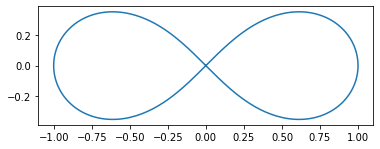We've loosely referred to the idea of inflating the zero-contour of a function $f(x, y)$ into 3D. The 3D function defining the desired implicit surface is

$$g(x, y, z) = f(x, y)^2 + z^2 - r^2,$$

where $r$ is a free parameter that we'll have to tune. I'm going to guess that $r < \frac{a}{\sqrt{2}}$ but it could be much less; beyond that we'll have to figure out what $r$ is by trial and error.

The code below uses the sympy software package to create a symbolic representation of the function $g$ defining our surface. Having a symbolic expression for $g$ allows us to evaluate it and its derivatives, but to actually visualize the surface we'll have to sample points on it somehow.

import sympy
x, y, z = sympy.symbols("x y z")
f = (x ** 2 + y ** 2) ** 2 - a ** 2 * (x ** 2 - y ** 2)

r = a / 6
g = f ** 2 + z ** 2 - r **2
dg = sympy.derive_by_array(g, [x, y, z])


Symbolically evaluating $g$ every time is expensive, so the code below uses the lambdify function from sympy to convert our symbolic expression into an ordinary Python function. I've added some additional wrappers so that we can pass in a numpy array of coordinates rather than the $x$, $y$, and $z$ values as separate arguments.

g_fn = sympy.lambdify([x, y, z], g, modules="numpy")
def G(q):
return np.array([g_fn(*q)])

dg_fn = sympy.lambdify([x, y, z], dg, modules="numpy")
def dG(q):
return np.array(dg_fn(*q)).reshape([1, 3])


One of the first algorithms for constrained mechanical systems was called SHAKE, so naturally some clever bastard had to make one called RATTLE and there's probably a ROLL out there too. The code below implements the RATTLE algorithm. You can view this as analogous to the Stormer-Verlet method, which does a half-step of the momentum solve, a full step of the position solve, and finally another half-step of the momentum solve again. In the RATTLE algorithm, we have to exercise a bit of foresight in the initial momentum half-step and position full-step in order to calculate a Lagrange multiplier to project an arbitrary position back onto the zero-contour of $g$. Solving for the position multiplier is a true nonlinear equation, whereas the final momentum half-step is just a linear equation for the momentum and its multiplier, which we've written here as $\mu$. Here we only have one constraint, so each multiplier is a scalar, which is a convenient simplification.

import tqdm
import scipy.linalg
import scipy.optimize

def trajectory(q, v, dt, num_steps, f, g, dg, progressbar=False):
qs = np.zeros((num_steps + 1,) + q.shape)
vs = np.zeros((num_steps + 1,) + q.shape)

g_0 = g(q)
λs = np.zeros((num_steps + 1,) + g_0.shape)
μs = np.zeros((num_steps + 1,) + g_0.shape)

def project_position(λ_0, q, v):
def fn(λ, q, v):
v_n = v + 0.5 * dt * (f(q) - λ @ dg(q))
q_n = q + dt * v_n
return g(q_n)

result = scipy.optimize.root(fn, λ_0, args=(q, v))
return result.x

def project_velocity(q, v):
J = dg(q)
# TODO: Don't solve the normal equations, you're making Anne G sad
A = J @ J.T
b = J @ v
return scipy.linalg.solve(A, b, assume_a="pos")

qs = q
μs = project_velocity(q, v)
vs = v - μs @ dg(q)

iterator = (tqdm.trange if progressbar else range)(num_steps)
for t in iterator:
λs[t + 1] = project_position(λs[t], qs[t], vs[t])
v_mid = vs[t] + 0.5 * dt * (f(qs[t]) - λs[t + 1] @ dg(qs[t]))
qs[t + 1] = qs[t] + dt * v_mid

v_prop = v_mid + 0.5 * dt * f(qs[t + 1])
μs[t + 1] = project_velocity(qs[t + 1], v_prop)
vs[t + 1] = v_mid + 0.5 * dt * f(qs[t + 1]) - μs[t + 1] @ dg(qs[t + 1])

return qs, vs, λs, μs


I'll add that this algorithm was exceedingly fiddly to implement and I had to debug about 5 or 6 times before I got it right. The sanity checking shown below was essential to making sure it was right.

def potential(q):
return q

def force(q):
return np.array((0, 0, -1))

num_trajectories = 25
θs = 2 * π * np.linspace(0, 1, num_trajectories)
num_steps = 2000
Qs = np.zeros((num_steps + 1, 3 * num_trajectories))
Vs = np.zeros((num_steps + 1, 3 * num_trajectories))
Λs = np.zeros((num_steps + 1, num_trajectories))
for i, θ in tqdm.tqdm(enumerate(θs), total=num_trajectories):
q = np.array((0, 0, r))
v = np.array((np.cos(θ), np.sin(θ), 0))
dt = 1e-2
qs, vs, λs, μs = trajectory(q, v, dt, num_steps, force, G, dG)
Qs[:, 3 * i : 3 * (i + 1)] = qs
Vs[:, 3 * i : 3 * (i + 1)] = vs
Λs[:, i] = λs.flatten()

100%|██████████| 25/25 [00:21<00:00,  1.16it/s]


As a sanity check, we'll evaluate the change in energy throughout the course of the simulation relative to the mean kinetic energy. The relative differences are on the order of 1%, which suggests that the method is doing a pretty good job. I re-ran this notebook with half the timestep and the energy deviation is cut by a factor of four, indicative of second-order convergence.

fig, ax = plt.subplots()
for i in range(num_trajectories):
qs, vs = Qs[:, 3 * i : 3 * (i + 1)], Vs[:, 3 * i : 3 * (i + 1)]
K = 0.5 * np.sum(vs ** 2, axis=1)
U = np.array([potential(q) for q in qs])
energies = K + U
ax.plot((energies - energies) / np.mean(K))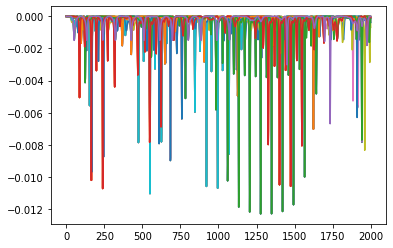Finally, let's make a movie of the results.

from mpl_toolkits import mplot3d
from mpl_toolkits.mplot3d.art3d import Line3DCollection
from matplotlib.animation import FuncAnimation

def make_animation(
Qs, depth=25, duration=30.0, start_width=0.1, end_width=1.5, ax=None
):
num_steps = Qs.shape
num_particles = Qs.shape // 3

widths = np.linspace(start_width, end_width, depth)
collections = []
for i in range(num_particles):
q_i = Qs[:depth, 3 * i : 3 * (i + 1)]
points = q_i.reshape(-1, 1, 3)
segments = np.concatenate([points[:-1], points[1:]], axis=1)
collection = Line3DCollection(segments, linewidths=widths)
collections.append(collection)

def update(step):
start = max(0, step - depth)
for i in range(num_particles):
q_i = Qs[step - depth : step, 3 * i : 3 * (i + 1)]
points = q_i.reshape(-1, 1, 3)
segments = np.concatenate([points[:-1], points[1:]], axis=1)
collections[i].set_segments(segments)

interval = 1e3 * duration / num_steps
frames = list(range(depth, num_steps))
return FuncAnimation(
ax.figure, update, frames=frames, interval=interval, blit=False
)


My Riemannian geometry kung fu is weak is but I think that the geodesic flow on this surface is ergodic (see these notes).

%%capture

fig = plt.figure()
ax.set_xlim((-a, a))
ax.set_ylim((-a, a))
ax.set_zlim((-a, a))
ax.set_axis_off()

animation = make_animation(Qs, depth=100, ax=ax)

from IPython.display import HTML
HTML(animation.to_html5_video())


It's also interesting to have a look at what the respective Lagrange multipliers for position and velocity are doing.

fig, ax = plt.subplots()
ts = np.linspace(0.0, num_steps * dt, num_steps + 1)
ax.plot(ts, Λs[:, 6].reshape(-1));